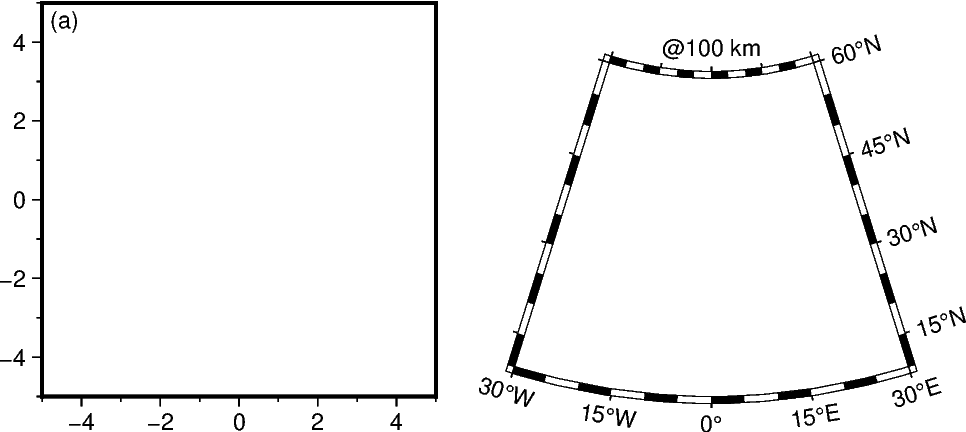# Plotting text

It is often useful to add annotations to a plot. This is handled by `pygmt.Figure.text`.

```import os

import pygmt
```

## Basic map annotation

Text annotations can be added to a map using the `pygmt.Figure.text` method of the `pygmt.Figure` class.

Here we create a simple map and add an annotation using the `text`, `x`, and `y` parameters to specify the annotation text and position in the projection frame. `text` accepts str types, while `x` and `y` accept either int or float numbers, or a list/array of numbers.

```fig = pygmt.Figure()
with pygmt.config(MAP_FRAME_TYPE="plain"):
fig.basemap(region=[108, 120, -5, 8], projection="M20c", frame="a")
fig.coast(land="black", water="skyblue")

# Plot text annotations using single arguments
fig.text(text="SOUTH CHINA SEA", x=112, y=6)

# Plot text annotations using lists of arguments
fig.text(text=["CELEBES SEA", "JAVA SEA"], x=[119, 112], y=[3.25, -4.6])

fig.show()
```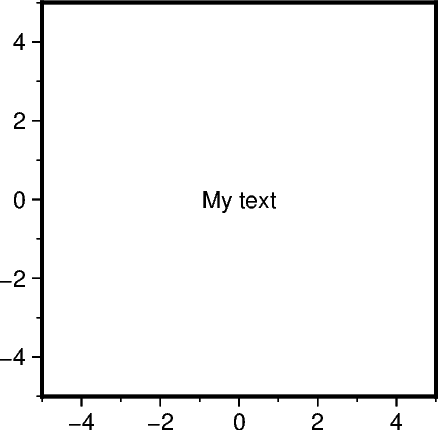## Changing font style

The size, family/weight, and color of an annotation can be specified using the `font` parameter.

A list of all recognized fonts can be found at PostScript Fonts Used by GMT, including details of how to use non-default fonts.

```fig = pygmt.Figure()
with pygmt.config(MAP_FRAME_TYPE="plain"):
fig.basemap(region=[108, 120, -5, 8], projection="M20c", frame="a")
fig.coast(land="black", water="skyblue")

# Customize the font style
fig.text(text="BORNEO", x=114.0, y=0.5, font="22p,Helvetica-Bold,white")

fig.show()
```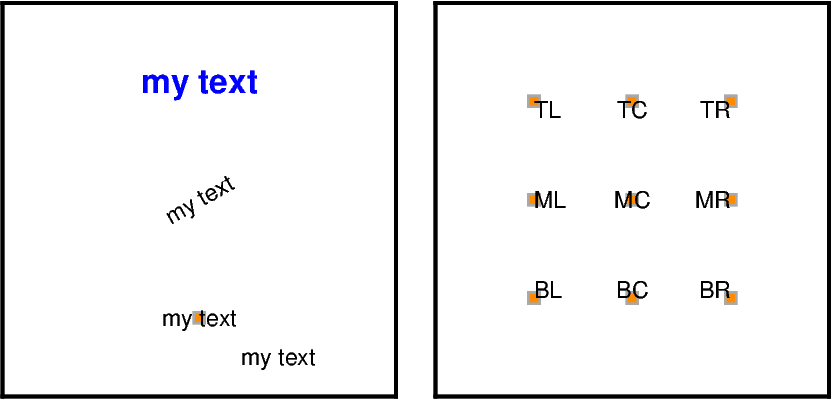## Plotting from a text file

It is also possible to add annotations from a file containing `x`, `y`, and `text` columns. Here we give a complete example.

```fig = pygmt.Figure()
with pygmt.config(MAP_FRAME_TYPE="plain"):
fig.basemap(region=[108, 120, -5, 8], projection="M20c", frame="a")
fig.coast(land="black", water="skyblue")

# Create space-delimited file
with open("examples.txt", "w") as f:
f.write("114 0.5 0 22p,Helvetica-Bold,white CM BORNEO\n")
f.write("119 3.25 0 12p,Helvetica-Bold,black CM CELEBES SEA\n")
f.write("112 -4.6 0 12p,Helvetica-Bold,black CM JAVA SEA\n")
f.write("112 6 40 12p,Helvetica-Bold,black CM SOUTH CHINA SEA\n")
f.write("119.12 7.25 -40 12p,Helvetica-Bold,black CM SULU SEA\n")
f.write("118.4 -1 65 12p,Helvetica-Bold,black CM MAKASSAR STRAIT\n")

# Plot region names / sea names from a text file, where
# the longitude (x) and latitude (y) coordinates are in the first two columns.
# Setting angle/font/justify to True will indicate that those columns are
# present in the text file too (Note: must be in that order!).
# Finally, the text to be printed will be in the last column
fig.text(textfiles="examples.txt", angle=True, font=True, justify=True)

# Cleanups
os.remove("examples.txt")

fig.show()
```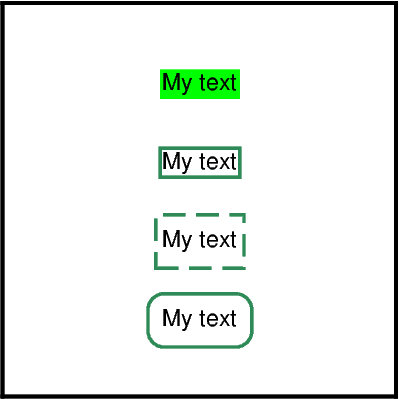## `justify` parameter

`justify` is used to define the anchor point for the bounding box for text being added to a plot. The following code segment demonstrates the positioning of the anchor point relative to the text.

The anchor point is specified with a two-letter (order independent) code, chosen from:

• Vertical anchor: T(op), M(iddle), B(ottom)

• Horizontal anchor: L(eft), C(entre), R(ight)

```fig = pygmt.Figure()
fig.basemap(region=[0, 3, 0, 3], projection="X10c", frame=["WSne", "af0.5g"])
for position in ("TL", "TC", "TR", "ML", "MC", "MR", "BL", "BC", "BR"):
fig.text(
text=position,
position=position,
font="28p,Helvetica-Bold,black",
justify=position,
)
fig.show()
```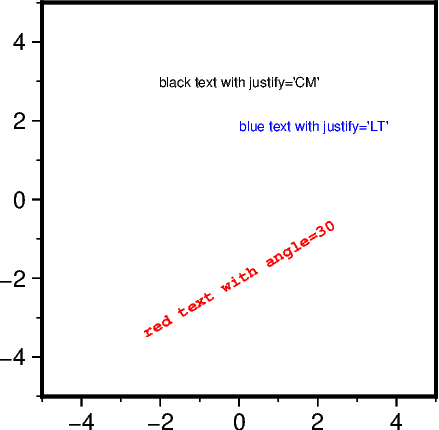## `angle` parameter

`angle` is an optional parameter used to specify the counter-clockwise rotation in degrees of the text from the horizontal.

```fig = pygmt.Figure()
fig.basemap(region=[0, 4, 0, 4], projection="X5c", frame="WSen")
for i in range(0, 360, 30):
fig.text(text=f"`          {i}@.", x=2, y=2, justify="LM", angle=i)
fig.show()
```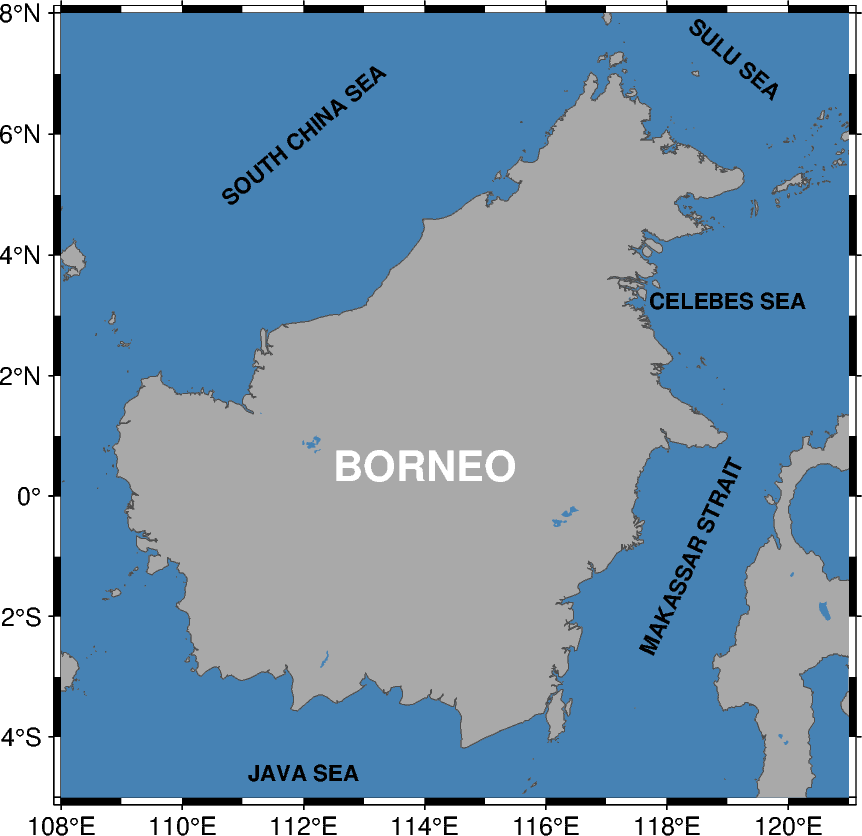## `fill` parameter

`fill` is used to set the fill color of the area surrounding the text.

```fig = pygmt.Figure()
fig.basemap(region=[0, 1, 0, 1], projection="X5c", frame="WSen")
fig.text(text="Green", x=0.5, y=0.5, fill="green")
fig.show()
```